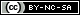# Weibull++ Examples

(Difference between revisions)
 Revision as of 03:12, 15 August 2012 (view source)← Older edit Revision as of 03:40, 15 August 2012 (view source) (→Test Design Examples)Newer edit → Line 45: Line 45: ==Test Design Examples== ==Test Design Examples== - * [[Parametric_Binomial_Example_-_Demonstrate_Reliability|Parametric Binomial Test Design Example - Test to Demonstrate Reliability]] + * [[Parametric_Binomial_Example_-_Demonstrate_Reliability|RDT: Parametric Binomial Test Design Example - Test to Demonstrate Reliability]] - * [[Parametric_Binomial_Example_-_Demonstrate_MTTF|Parametric Binomial Test Design Example - Test to Demonstrate MTTF]] + * [[Parametric_Binomial_Example_-_Demonstrate_MTTF|RDT: Parametric Binomial Test Design Example - Test to Demonstrate MTTF]] - * [[Non-Parametric Binomial Test Design Example]] + * [[Non-Parametric Binomial Test Design Example|RDT: Non-Parametric Binomial Test Design Example]] - * [[Exponential_Chi-Squared_Example| Exponential Chi-Squared Test Design Example]] + * [[Exponential_Chi-Squared_Example|RDT: Exponential Chi-Squared Test Design Example]] * [[Non-Parametric Bayesian - Expert Opinion|RDT: Non-Parametric Bayesian with Prior Information from Expert Opinion]] * [[Non-Parametric Bayesian - Expert Opinion|RDT: Non-Parametric Bayesian with Prior Information from Expert Opinion]] * [[Non-Parametric Bayesian - Subsystem Tests|RDT: Non-Parametric Bayesian with Prior Information from Subsystem Tests]] * [[Non-Parametric Bayesian - Subsystem Tests|RDT: Non-Parametric Bayesian with Prior Information from Subsystem Tests]]

## Life Data Analysis

• Crack Propagation Example (Point Estimation)
View it in HTML or Video

## Recurrent Event Data Analysis

View it in HTML or Video

## Warranty Data Analysis

View it in HTML or Video

## UtilitiesContent on this site is available/licensed under the "Creative Commons Attribution-NonCommercial-ShareAlike 4.0 International License." See Copyright Information for details.